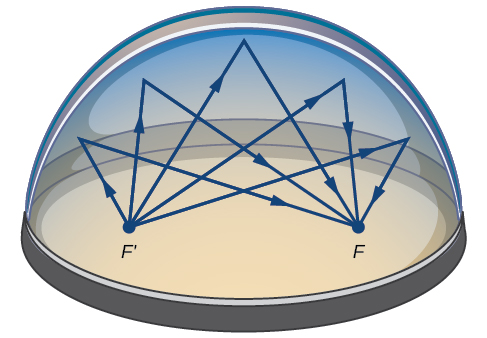# 12.1 The ellipse  (Page 7/16)

 Page 7 / 16

## Graphing an ellipse centered at ( h , k ) by first writing it in standard form

Graph the ellipse given by the equation $\text{\hspace{0.17em}}4{x}^{2}+9{y}^{2}-40x+36y+100=0.\text{\hspace{0.17em}}$ Identify and label the center, vertices, co-vertices, and foci.

We must begin by rewriting the equation in standard form.

$4{x}^{2}+9{y}^{2}-40x+36y+100=0$

Group terms that contain the same variable, and move the constant to the opposite side of the equation.

$\left(4{x}^{2}-40x\right)+\left(9{y}^{2}+36y\right)=-100$

Factor out the coefficients of the squared terms.

$4\left({x}^{2}-10x\right)+9\left({y}^{2}+4y\right)=-100$

Complete the square twice. Remember to balance the equation by adding the same constants to each side.

$4\left({x}^{2}-10x+25\right)+9\left({y}^{2}+4y+4\right)=-100+100+36$

Rewrite as perfect squares.

$4{\left(x-5\right)}^{2}+9{\left(y+2\right)}^{2}=36$

Divide both sides by the constant term to place the equation in standard form.

$\frac{{\left(x-5\right)}^{2}}{9}+\frac{{\left(y+2\right)}^{2}}{4}=1$

Now that the equation is in standard form, we can determine the position of the major axis. Because $\text{\hspace{0.17em}}9>4,\text{\hspace{0.17em}}$ the major axis is parallel to the x -axis. Therefore, the equation is in the form $\text{\hspace{0.17em}}\frac{{\left(x-h\right)}^{2}}{{a}^{2}}+\frac{{\left(y-k\right)}^{2}}{{b}^{2}}=1,\text{\hspace{0.17em}}$ where $\text{\hspace{0.17em}}{a}^{2}=9\text{\hspace{0.17em}}$ and $\text{\hspace{0.17em}}{b}^{2}=4.\text{\hspace{0.17em}}$ It follows that:

• the center of the ellipse is $\text{\hspace{0.17em}}\left(h,k\right)=\left(5,-2\right)$
• the coordinates of the vertices are $\text{\hspace{0.17em}}\left(h±a,k\right)=\left(5±\sqrt{9},-2\right)=\left(5±3,-2\right),\text{\hspace{0.17em}}$ or $\text{\hspace{0.17em}}\left(2,-2\right)\text{\hspace{0.17em}}$ and $\text{\hspace{0.17em}}\left(8,-2\right)$
• the coordinates of the co-vertices are $\text{\hspace{0.17em}}\left(h,k±b\right)=\left(\text{5},-2±\sqrt{4}\right)=\left(\text{5},-2±2\right),\text{\hspace{0.17em}}$ or $\text{\hspace{0.17em}}\left(5,-4\right)\text{\hspace{0.17em}}$ and $\text{\hspace{0.17em}}\left(5,\text{0}\right)$
• the coordinates of the foci are $\text{\hspace{0.17em}}\left(h±c,k\right),\text{\hspace{0.17em}}$ where $\text{\hspace{0.17em}}{c}^{2}={a}^{2}-{b}^{2}.\text{\hspace{0.17em}}$ Solving for $\text{\hspace{0.17em}}c,\text{\hspace{0.17em}}$ we have:
$\begin{array}{l}c=±\sqrt{{a}^{2}-{b}^{2}}\hfill \\ \text{\hspace{0.17em}}\text{\hspace{0.17em}}\text{\hspace{0.17em}}=±\sqrt{9-4}\hfill \\ \text{\hspace{0.17em}}\text{\hspace{0.17em}}\text{\hspace{0.17em}}=±\sqrt{5}\hfill \end{array}$

Therefore, the coordinates of the foci are $\text{\hspace{0.17em}}\left(\text{5}-\sqrt{5},-2\right)\text{\hspace{0.17em}}$ and $\text{\hspace{0.17em}}\left(\text{5+}\sqrt{5},-2\right).$

Next we plot and label the center, vertices, co-vertices, and foci, and draw a smooth curve to form the ellipse as shown in [link] .

Express the equation of the ellipse given in standard form. Identify the center, vertices, co-vertices, and foci of the ellipse.

$4{x}^{2}+{y}^{2}-24x+2y+21=0$

$\text{\hspace{0.17em}}\frac{{\left(x-3\right)}^{2}}{4}+\frac{{\left(y+1\right)}^{2}}{16}=1;\text{\hspace{0.17em}}$ center: $\text{\hspace{0.17em}}\left(3,-1\right);\text{\hspace{0.17em}}$ vertices: $\text{\hspace{0.17em}}\left(3,-\text{5}\right)\text{\hspace{0.17em}}$ and $\text{\hspace{0.17em}}\left(3,\text{3}\right);\text{\hspace{0.17em}}$ co-vertices: $\text{\hspace{0.17em}}\left(1,-1\right)\text{\hspace{0.17em}}$ and $\text{\hspace{0.17em}}\left(5,-1\right);\text{\hspace{0.17em}}$ foci: $\text{\hspace{0.17em}}\left(3,-\text{1}-2\sqrt{3}\right)\text{\hspace{0.17em}}$ and $\text{\hspace{0.17em}}\left(3,-\text{1+}2\sqrt{3}\right)$

## Solving applied problems involving ellipses

Many real-world situations can be represented by ellipses, including orbits of planets, satellites, moons and comets, and shapes of boat keels, rudders, and some airplane wings. A medical device called a lithotripter uses elliptical reflectors to break up kidney stones by generating sound waves. Some buildings, called whispering chambers, are designed with elliptical domes so that a person whispering at one focus can easily be heard by someone standing at the other focus. This occurs because of the acoustic properties of an ellipse. When a sound wave originates at one focus of a whispering chamber, the sound wave will be reflected off the elliptical dome and back to the other focus. See [link] . In the whisper chamber at the Museum of Science and Industry in Chicago, two people standing at the foci—about 43 feet apart—can hear each other whisper.Sound waves are reflected between foci in an elliptical room, called a whispering chamber.

## Locating the foci of a whispering chamber

The Statuary Hall in the Capitol Building in Washington, D.C. is a whispering chamber. Its dimensions are 46 feet wide by 96 feet long as shown in [link] .

1. What is the standard form of the equation of the ellipse representing the outline of the room? Hint: assume a horizontal ellipse, and let the center of the room be the point $\text{\hspace{0.17em}}\left(0,0\right).$
2. If two senators standing at the foci of this room can hear each other whisper, how far apart are the senators? Round to the nearest foot.
1. We are assuming a horizontal ellipse with center $\text{\hspace{0.17em}}\left(0,0\right),$ so we need to find an equation of the form $\text{\hspace{0.17em}}\frac{{x}^{2}}{{a}^{2}}+\frac{{y}^{2}}{{b}^{2}}=1,\text{\hspace{0.17em}}$ where $\text{\hspace{0.17em}}a>b.\text{\hspace{0.17em}}$ We know that the length of the major axis, $\text{\hspace{0.17em}}2a,\text{\hspace{0.17em}}$ is longer than the length of the minor axis, $\text{\hspace{0.17em}}2b.\text{\hspace{0.17em}}$ So the length of the room, 96, is represented by the major axis, and the width of the room, 46, is represented by the minor axis.
• Solving for $\text{\hspace{0.17em}}a,$ we have $\text{\hspace{0.17em}}2a=96,$ so $\text{\hspace{0.17em}}a=48,$ and $\text{\hspace{0.17em}}{a}^{2}=2304.$
• Solving for $\text{\hspace{0.17em}}b,$ we have $\text{\hspace{0.17em}}2b=46,$ so $\text{\hspace{0.17em}}b=23,$ and $\text{\hspace{0.17em}}{b}^{2}=529.$

Therefore, the equation of the ellipse is $\text{\hspace{0.17em}}\frac{{x}^{2}}{2304}+\frac{{y}^{2}}{529}=1.$

2. To find the distance between the senators, we must find the distance between the foci, $\text{\hspace{0.17em}}\left(±c,0\right),\text{\hspace{0.17em}}$ where $\text{\hspace{0.17em}}{c}^{2}={a}^{2}-{b}^{2}.\text{\hspace{0.17em}}$ Solving for $\text{\hspace{0.17em}}c,$ we have:

The points $\text{\hspace{0.17em}}\left(±42,0\right)\text{\hspace{0.17em}}$ represent the foci. Thus, the distance between the senators is $\text{\hspace{0.17em}}2\left(42\right)=84\text{\hspace{0.17em}}$ feet.

A laser rangefinder is locked on a comet approaching Earth. The distance g(x), in kilometers, of the comet after x days, for x in the interval 0 to 30 days, is given by g(x)=250,000csc(π30x). Graph g(x) on the interval [0, 35]. Evaluate g(5)  and interpret the information. What is the minimum distance between the comet and Earth? When does this occur? To which constant in the equation does this correspond? Find and discuss the meaning of any vertical asymptotes.
The sequence is {1,-1,1-1.....} has
how can we solve this problem
Sin(A+B) = sinBcosA+cosBsinA
Prove it
Eseka
Eseka
hi
Joel
June needs 45 gallons of punch. 2 different coolers. Bigger cooler is 5 times as large as smaller cooler. How many gallons in each cooler?
7.5 and 37.5
Nando
find the sum of 28th term of the AP 3+10+17+---------
I think you should say "28 terms" instead of "28th term"
Vedant
the 28th term is 175
Nando
192
Kenneth
if sequence sn is a such that sn>0 for all n and lim sn=0than prove that lim (s1 s2............ sn) ke hole power n =n
write down the polynomial function with root 1/3,2,-3 with solution
if A and B are subspaces of V prove that (A+B)/B=A/(A-B)
write down the value of each of the following in surd form a)cos(-65°) b)sin(-180°)c)tan(225°)d)tan(135°)
Prove that (sinA/1-cosA - 1-cosA/sinA) (cosA/1-sinA - 1-sinA/cosA) = 4
what is the answer to dividing negative index
In a triangle ABC prove that. (b+c)cosA+(c+a)cosB+(a+b)cisC=a+b+c.
give me the waec 2019 questionsByByBy Anonymous User